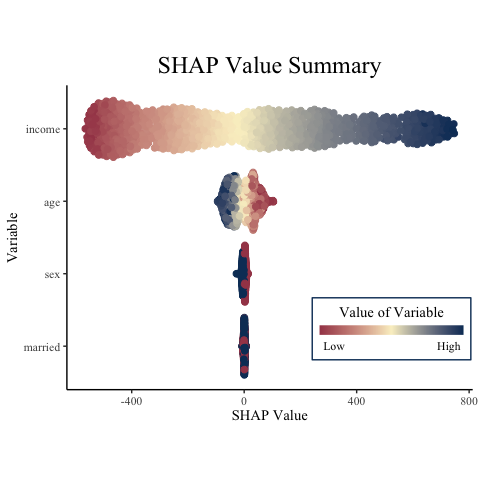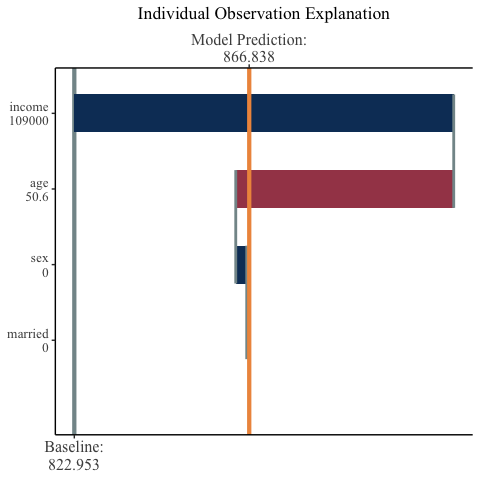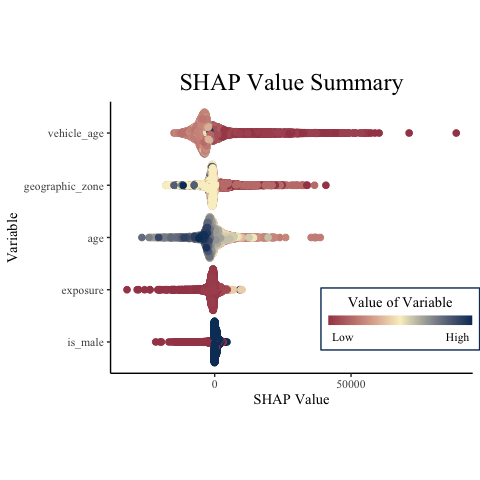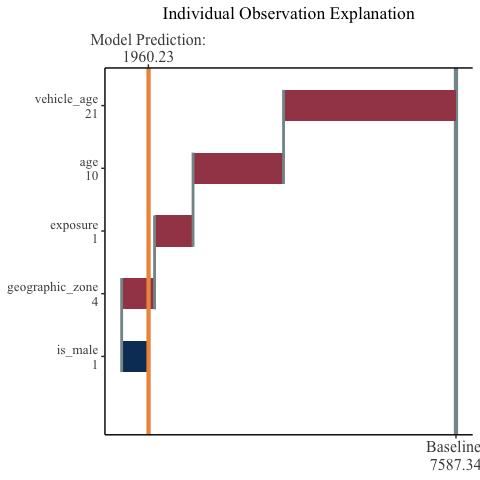# mSHAP

## Introduction

The purpose of this vignette will be to explore different use cases for mSHAP. It will focus heavily on insurance ratemaking, and will be based on the “AutoClaims” and “dataOhlsson” data sets that can be obtained in the {insuranceData} package, as demonstrated below:

## R
library(mshap)
library(reticulate) # for accessing python objects from r and vice-versa
#> Warning: package 'reticulate' was built under R version 4.0.2
library(insuranceData) # for the data
#> Warning: package 'insuranceData' was built under R version 4.0.2
library(dplyr) # for the data manipulation
#> Warning: package 'dplyr' was built under R version 4.0.2
#>
#> Attaching package: 'dplyr'
#> The following objects are masked from 'package:stats':
#>
#>     filter, lag
#> The following objects are masked from 'package:base':
#>
#>     intersect, setdiff, setequal, union
library(purrr) # for mapping over lists returned from python
library(caret) # for train/test split
#> Warning: package 'caret' was built under R version 4.0.2
#>
#> Attaching package: 'caret'
#> The following object is masked from 'package:purrr':
#>
#>     lift
data("dataOhlsson") # the data we will use for the second example

# If you do not havae the needed python modules, uncomment and run the code below:
# if (!py_module_available("numpy")) py_install("numpy", pip = TRUE)
# if (!py_module_available("pandas")) py_install("pandas", pip = TRUE)
# if (!py_module_available("shap")) py_install("shap", pip = TRUE)
# if (!py_module_available("sklearn")) py_install("sklearn", pip = TRUE)

In addition to the R libraries included above, we will need the additional python modules for these examples:

## Python
import numpy as np
import pandas as pd
import shap
import sklearn.ensemble as sk

Numpy and Pandas are for data manipulation, shap allows us to calculate the SHAP values using TreeSHAP, and sklearn.ensemble is necessary as the models we will create will be random forest models using scikit-learn.

## Basic Use Case

First, we will demonstrate a simple use case on simulated data. Suppose that we wish to be able to predict to total amount of money a consumer will spend on a subscription to a software product. We might simulate 4 explanatory variables that looks like the following:

## R
set.seed(16)
age <- runif(1000, 18, 60)
income <- runif(1000, 50000, 150000)
married <- as.numeric(runif(1000, 0, 1) > 0.5)
sex <- as.numeric(runif(1000, 0, 1) > 0.5)
# For the sake of simplicity we will have these as numeric already, where 0 represents male and 1 represents female

Now because this is a contrived example, we will knowingly set the response variables as follows (suppose here that cost_per_month is usage based, so as to be continuous):

## R
cost_per_month <- (0.0006 * income - 0.2 * sex + 0.5 * married - 0.001 * age) + 10
num_months <- (0.0001 * income + 0.0001 * sex + 0.05 * married - 0.05 * age) + 3

Thus, we have our data. We will combine the covariates into a single data frame for ease of use in python.

## R
X <- data.frame(age, income, married, sex)

The end goal of this exercise is to predict the total revenue from the given customer, which mathematically will be cost_per_month * num_months. Instead of multiplying these two vectors together initially, we will instead create two models: one to predict cost_per_month and the other to predict num_months. We can then multiply the output of the two models together to get our predictions.

We now move over to python to create our two models and predict on the training sets:

## Python
X = r.X
y1 = r.cost_per_month
y2 = r.num_months

cpm_mod = sk.RandomForestRegressor(n_estimators = 100, max_depth = 10, max_features = 2)
cpm_mod.fit(X, y1)
#> RandomForestRegressor(max_depth=10, max_features=2)
nm_mod = sk.RandomForestRegressor(n_estimators = 100, max_depth = 10, max_features = 2)
nm_mod.fit(X, y2)
#> RandomForestRegressor(max_depth=10, max_features=2)
cpm_preds = cpm_mod.predict(X)
nm_preds = nm_mod.predict(X)

tot_rev = cpm_preds * nm_preds

We will now proceed to use TreeSHAP and subsequently mSHAP to explain the ultimate model predictions.

## Python

# because these are tree-based models, shap.Explainer uses TreeSHAP to calculate
# fast, exact SHAP values for each model individually
cpm_ex = shap.Explainer(cpm_mod)
cpm_shap = cpm_ex.shap_values(X)
cpm_expected_value = cpm_ex.expected_value

nm_ex = shap.Explainer(nm_mod)
nm_shap = nm_ex.shap_values(X)
nm_expected_value = nm_ex.expected_value

## R
final_shap <- mshap(
shap_1 = py$cpm_shap, shap_2 = py$nm_shap,
ex_1 = py$cpm_expected_value, ex_2 = py$nm_expected_value
)

head(final_shap$shap_vals) #> # A tibble: 6 x 4 #> V1 V2 V3 V4 #> <dbl> <dbl> <dbl> <dbl> #> 1 -28.8 -375. -2.52 -4.52 #> 2 48.9 629. 3.10 -6.41 #> 3 6.16 533. 1.54 4.25 #> 4 29.2 -435. -0.444 -4.91 #> 5 -71.0 585. 0.138 -5.44 #> 6 31.3 419. -0.868 -7.11 final_shap$expected_value
#>  822.9525

As a check, you can see that the expected value for mSHAP is indeed the expected value of the model across the training data.

## R
mean(py$tot_rev) #>  822.9525 We now have calculated the mSHAP values for the multiplied model outputs! This will allow us to explain our final model. The mSHAP package comes with additional functions that can be used to visualize SHAP values in R. What is show here are the default outputs, but these functions return {ggplot2} objects that are easily customizable. ## R summary_plot( variable_values = X, shap_values = final_shap$shap_vals,
names = c("age", "income", "married", "sex") # this is optional, since X has column names
)## R
observation_plot(
variable_values = X[46,],
shap_values = final_shap$shap_vals[46,], expected_value = final_shap$expected_value,
names = c("age", "income", "married", "sex")
)
#> Warning in min(x): no non-missing arguments to min; returning Inf
#> Warning in max(x): no non-missing arguments to max; returning -Inf## Use Case on Ohlsson Data

We will now work through a little bit of a different use case, one specific to the insurance industry. In it, we will create a two-part model to predict the ultimate cost of the policy by using the first part of the model to predict the severity and the second part of the model to predict the frequency. Our frequency model will be a multinomial model, which will allow us to demonstrate using mSHAP with a multinomial output.

### Step 1: Prepare the Data

Let’s take a look at the data we will be using.

## R
#> 1       0   M   1       4      12       1 0.175342       0        0
#> 2       4   M   3       6       9       1 0.000000       0        0
#> 3       5   K   3       3      18       1 0.454795       0        0
#> 4       5   K   4       1      25       1 0.172603       0        0
#> 5       6   K   2       1      26       1 0.180822       0        0
#> 6       9   K   3       3       8       1 0.542466       0        0

We will first rename the columns, and look at summaries of each of the variables. Scikit-learn does not accept non-numeric covariates, so we will also convert the gender variable to an is_male indicator.

## R
cleaned <- dataOhlsson %>%
select(
severity,
exposure = duration,
age = agarald,
gender = kon,
geographic_zone = zon,
vehicle_age = fordald
) %>%
mutate(is_male = as.numeric(gender == "M")) %>%
select(-gender)

summary(cleaned$severity) #> Min. 1st Qu. Median Mean 3rd Qu. Max. NA's #> 16 3008 8724 23793 26788 211254 63878 summary(cleaned$exposure)
#>    Min. 1st Qu.  Median    Mean 3rd Qu.    Max.
#>  0.0000  0.4630  0.8274  1.0107  1.0000 31.3397
summary(cleaned$age) #> Min. 1st Qu. Median Mean 3rd Qu. Max. #> 0.00 31.00 44.00 42.42 52.00 92.00 summary(cleaned$vehicle_age)
#>    Min. 1st Qu.  Median    Mean 3rd Qu.    Max.
#>    0.00    5.00   12.00   12.54   16.00   99.00
cleaned %>% count(is_male)
#>   is_male     n
#> 1       0  9853
#> 2       1 54695
cleaned %>% count(geographic_zone)
#>   geographic_zone     n
#> 1               1  8582
#> 2               2 11794
#> 3               3 12722
#> 4               4 24816
#> 5               5  2377
#> 6               6  3884
#> 7               7   373

Our next step will be to create a train/test split on the overall data. This will allow us to train models with the train set while having a holdout set for prediction and explanation. We will use 90% of our data to train the model and only 10% to test it, for faster run times on the SHAP explainers.

## R
preds_freq <- py$preds_freq %>% as.data.frame() expected_values <- map2_dfc( .x = preds_freq, .y = 0:2, .f = ~{ .x * .y * preds_sev } ) %>% rowSums() ### Step 4: Explain the Predictions With the goal of explaining this “average value” prediction, we will eventually use mSHAP. However, it is necessary to first calculate the SHAP values for the two models separately. ## Python sev_ex = shap.Explainer(sev_mod) sev_expected_val = sev_ex.expected_value sev_preds_explained = sev_ex.shap_values(test_sev) freq_ex = shap.Explainer(freq_mod) freq_expected_val = freq_ex.expected_value freq_preds_explained = freq_ex.shap_values(test_freq) ## R freq_shap <- py$freq_preds_explained
sev_shap <- py$sev_preds_explained Note that we can take these raw SHAP values from python and plug them straight into the function with no additional manipulation, but that requires that we specify the arguments shap_1_names and shap_2_names. Recall that our models do not use exactly the same predictors, so specifying the names will alert the algorithm of this and create a column of zeros for all non-matching column names. Note also that passing in a list as one of the shap* arguments will cause a nested list to be returned, instead of the normal list. ## R mshap_res <- mshap( shap_1 = freq_shap, shap_2 = sev_shap, ex_1 = py$freq_expected_val,
ex_2 = py$sev_expected_val, shap_1_names = colnames(test_freq), shap_2_names = colnames(test_sev) ) Since we want the expected value, we would like to combine the SHAP values in the same way, multiplying the respective values by 0, 1, and 2. This can be done in the following code. ## R ev_explained <- mshap_res[]$shap_vals * 0 +
mshap_res[]$shap_vals * 1 + mshap_res[]$shap_vals * 2

shap_expected_values <- mshap_res[]$expected_value * 0 + mshap_res[]$expected_value * 1 +
mshap_res[]\$expected_value * 2

### Step 5: Visualize the Results

## R
summary_plot(variable_values = test_freq, shap_values = ev_explained)## R
observation_plot(variable_values = test_freq[1,], shap_values = ev_explained[1,], expected_value = shap_expected_values)
#> Warning in min(x): no non-missing arguments to min; returning Inf
#> Warning in max(x): no non-missing arguments to max; returning -Inf## Conclusion

Overall, mSHAP can be a great help when working with models where the ultimate prediction is the product of two different models, as is the case in the classic two-part model in the insurance industry.